Скачать презентацию Text Categorization 1 Categorization Given

88b4e1447eaa60e20324ef1113ff7316.ppt

• Количество слайдов: 74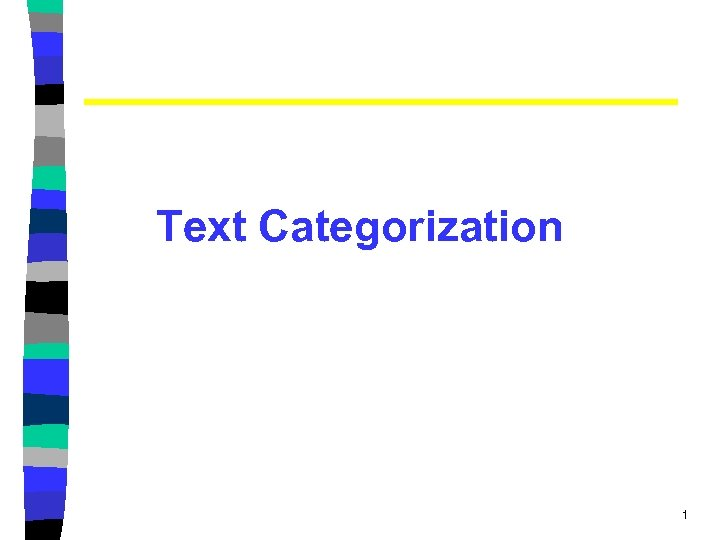Text Categorization 1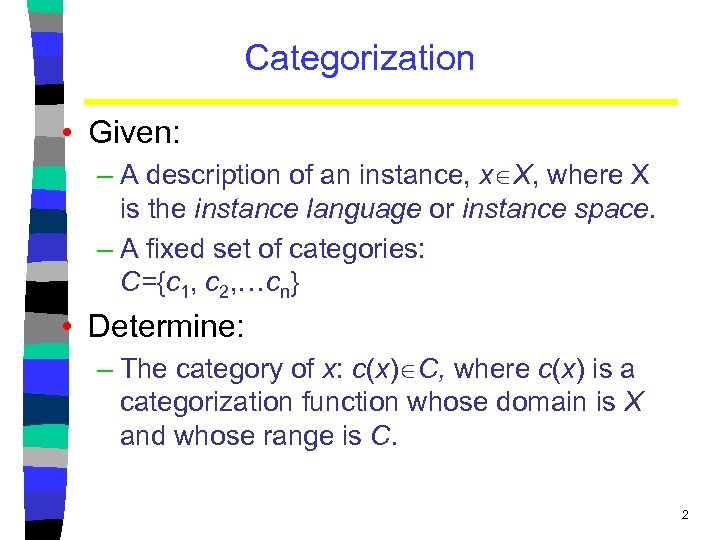Categorization • Given: – A description of an instance, x X, where X is the instance language or instance space. – A fixed set of categories: C={c 1, c 2, …cn} • Determine: – The category of x: c(x) C, where c(x) is a categorization function whose domain is X and whose range is C. 2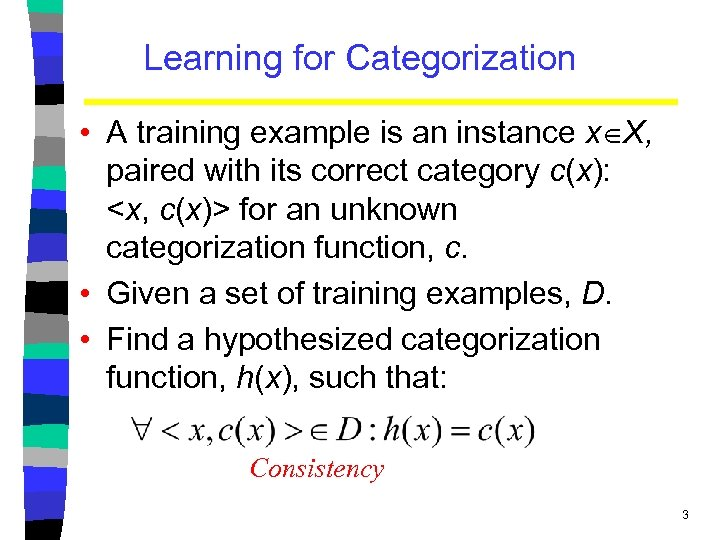Learning for Categorization • A training example is an instance x X, paired with its correct category c(x): for an unknown categorization function, c. • Given a set of training examples, D. • Find a hypothesized categorization function, h(x), such that: Consistency 3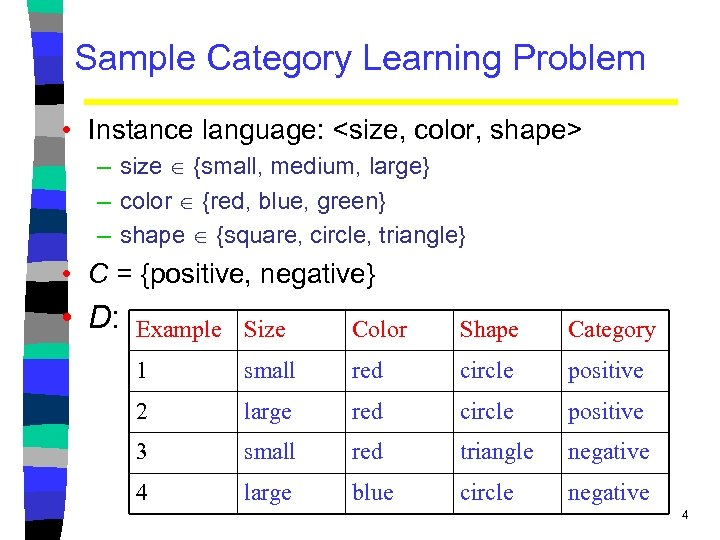Sample Category Learning Problem • Instance language: – size {small, medium, large} – color {red, blue, green} – shape {square, circle, triangle} • C = {positive, negative} • D: Example Size Color Shape Category 1 small red circle positive 2 large red circle positive 3 small red triangle negative 4 large blue circle negative 4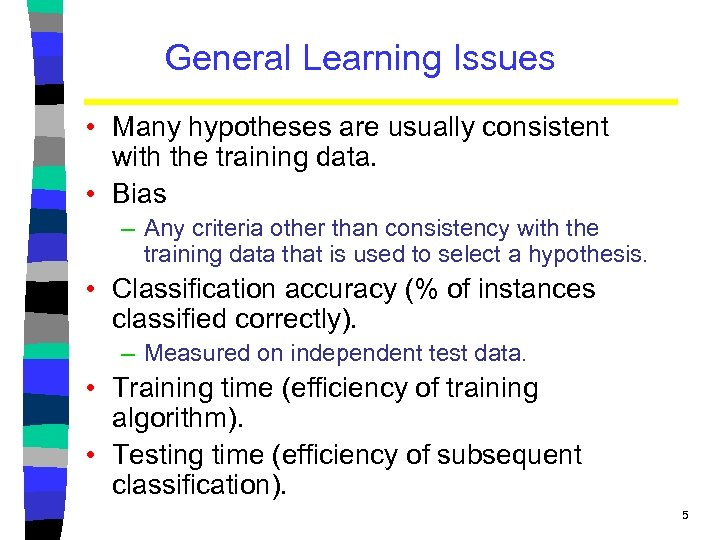General Learning Issues • Many hypotheses are usually consistent with the training data. • Bias – Any criteria other than consistency with the training data that is used to select a hypothesis. • Classification accuracy (% of instances classified correctly). – Measured on independent test data. • Training time (efficiency of training algorithm). • Testing time (efficiency of subsequent classification). 5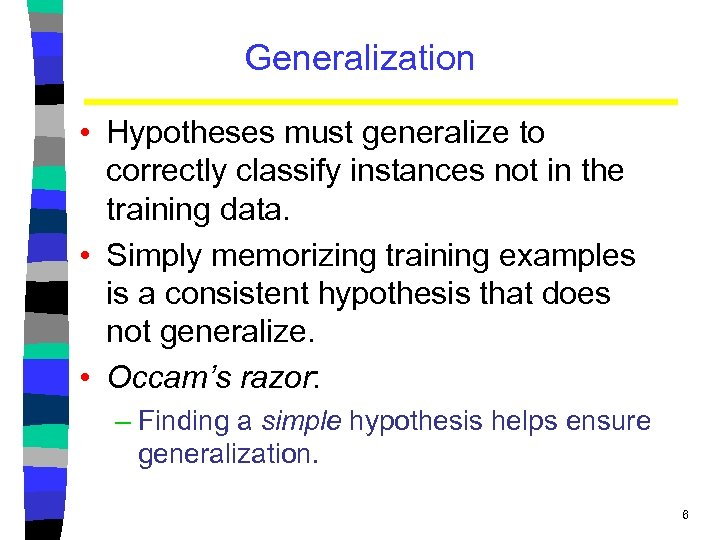Generalization • Hypotheses must generalize to correctly classify instances not in the training data. • Simply memorizing training examples is a consistent hypothesis that does not generalize. • Occam’s razor: – Finding a simple hypothesis helps ensure generalization. 6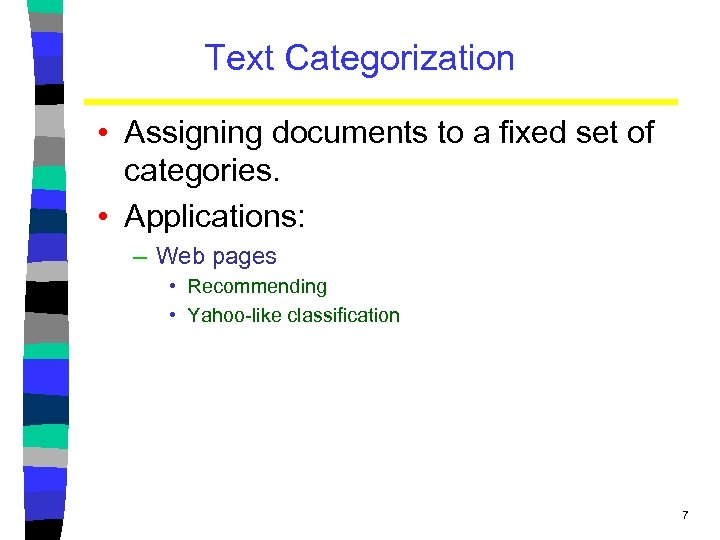Text Categorization • Assigning documents to a fixed set of categories. • Applications: – Web pages • Recommending • Yahoo-like classification 7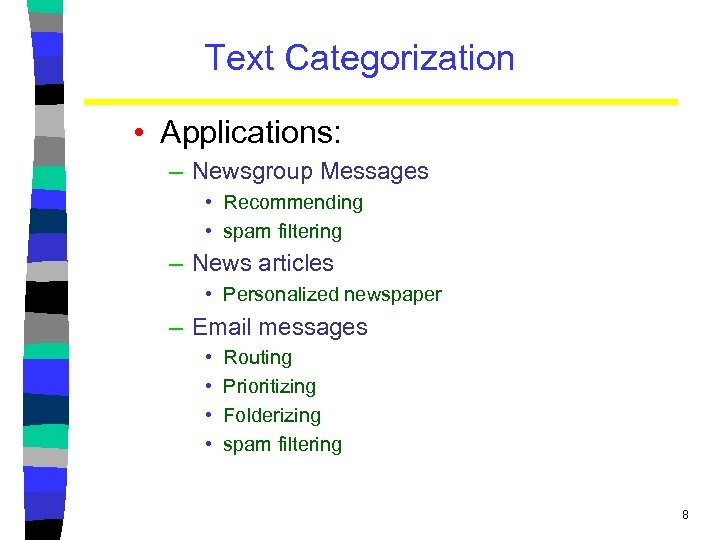Text Categorization • Applications: – Newsgroup Messages • Recommending • spam filtering – News articles • Personalized newspaper – Email messages • • Routing Prioritizing Folderizing spam filtering 8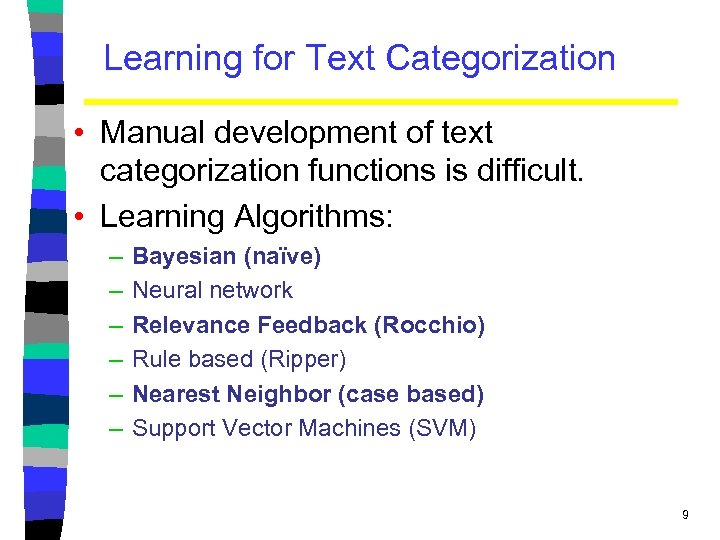Learning for Text Categorization • Manual development of text categorization functions is difficult. • Learning Algorithms: – – – Bayesian (naïve) Neural network Relevance Feedback (Rocchio) Rule based (Ripper) Nearest Neighbor (case based) Support Vector Machines (SVM) 9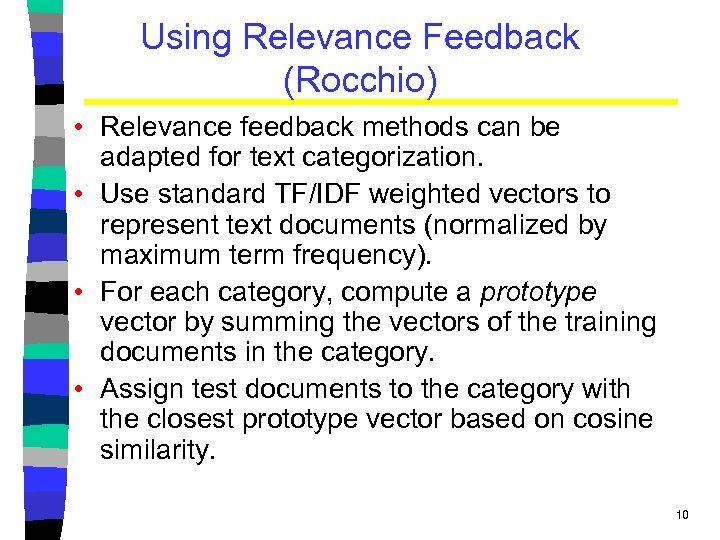Using Relevance Feedback (Rocchio) • Relevance feedback methods can be adapted for text categorization. • Use standard TF/IDF weighted vectors to represent text documents (normalized by maximum term frequency). • For each category, compute a prototype vector by summing the vectors of the training documents in the category. • Assign test documents to the category with the closest prototype vector based on cosine similarity. 10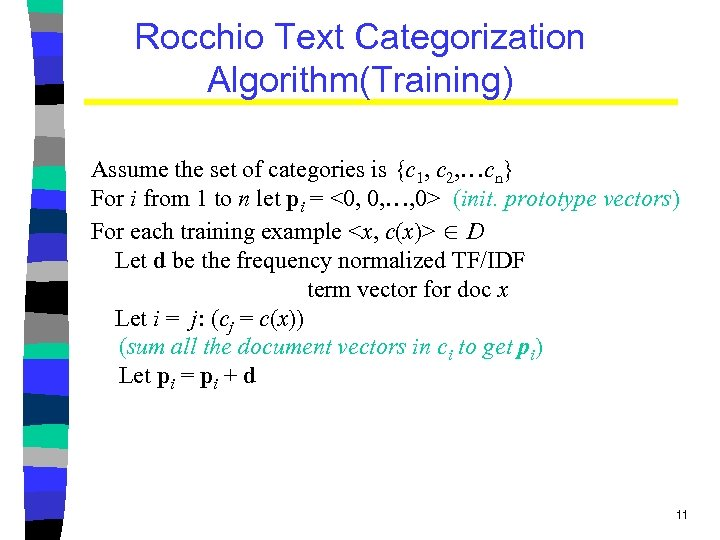Rocchio Text Categorization Algorithm(Training) Assume the set of categories is {c 1, c 2, …cn} For i from 1 to n let pi = <0, 0, …, 0> (init. prototype vectors) For each training example D Let d be the frequency normalized TF/IDF term vector for doc x Let i = j: (cj = c(x)) (sum all the document vectors in ci to get pi) Let pi = pi + d 11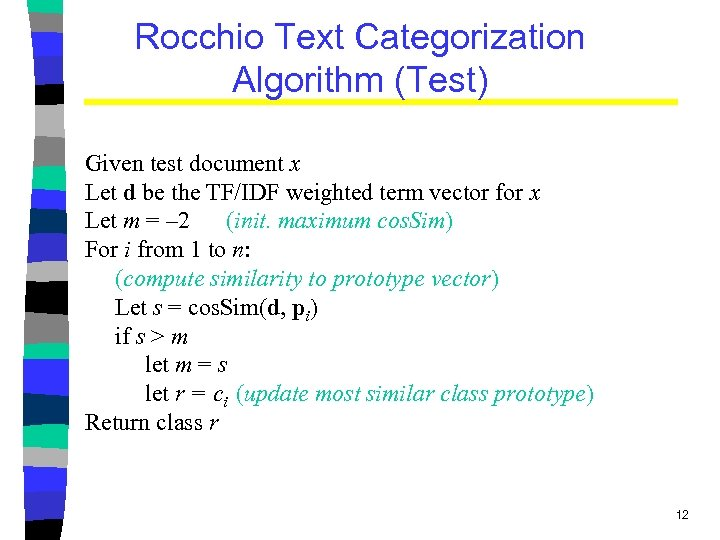Rocchio Text Categorization Algorithm (Test) Given test document x Let d be the TF/IDF weighted term vector for x Let m = – 2 (init. maximum cos. Sim) For i from 1 to n: (compute similarity to prototype vector) Let s = cos. Sim(d, pi) if s > m let m = s let r = ci (update most similar class prototype) Return class r 12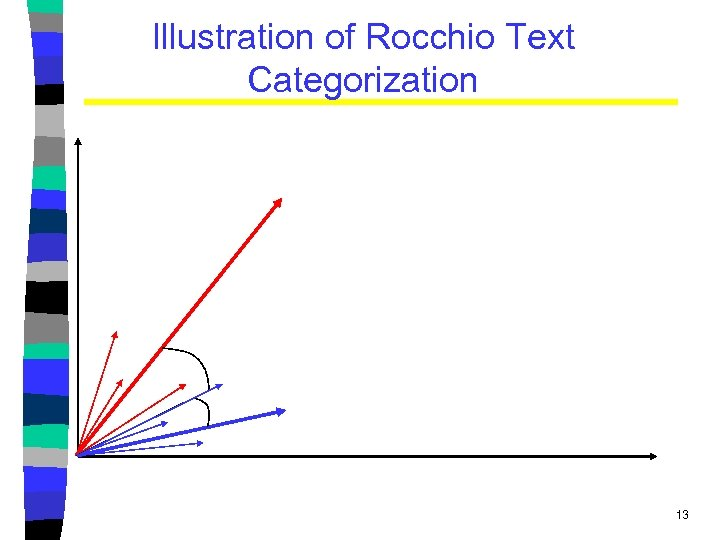Illustration of Rocchio Text Categorization 13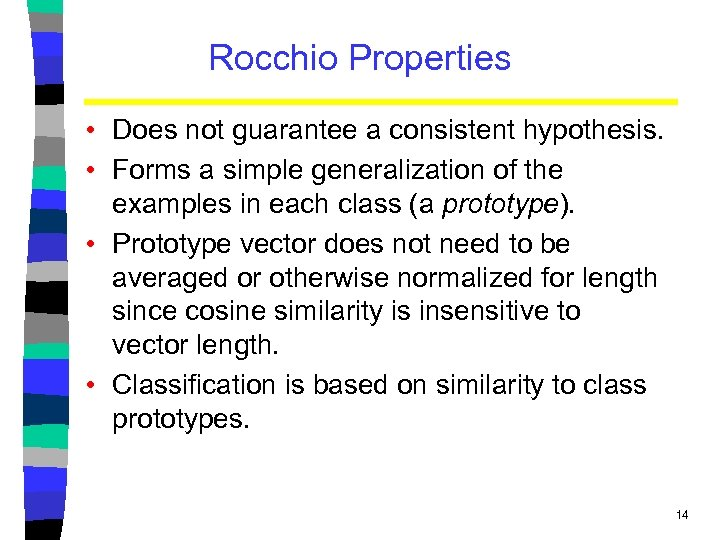Rocchio Properties • Does not guarantee a consistent hypothesis. • Forms a simple generalization of the examples in each class (a prototype). • Prototype vector does not need to be averaged or otherwise normalized for length since cosine similarity is insensitive to vector length. • Classification is based on similarity to class prototypes. 14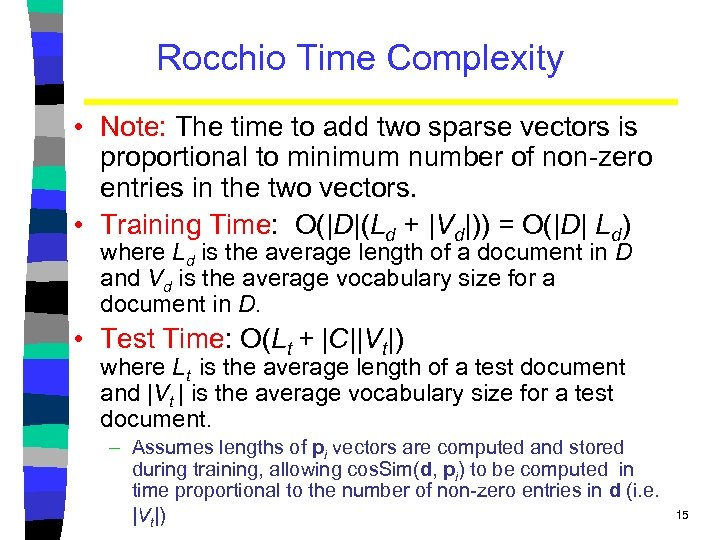Rocchio Time Complexity • Note: The time to add two sparse vectors is proportional to minimum number of non-zero entries in the two vectors. • Training Time: O(|D|(Ld + |Vd|)) = O(|D| Ld) where Ld is the average length of a document in D and Vd is the average vocabulary size for a document in D. • Test Time: O(Lt + |C||Vt|) where Lt is the average length of a test document and |Vt | is the average vocabulary size for a test document. – Assumes lengths of pi vectors are computed and stored during training, allowing cos. Sim(d, pi) to be computed in time proportional to the number of non-zero entries in d (i. e. |Vt|) 15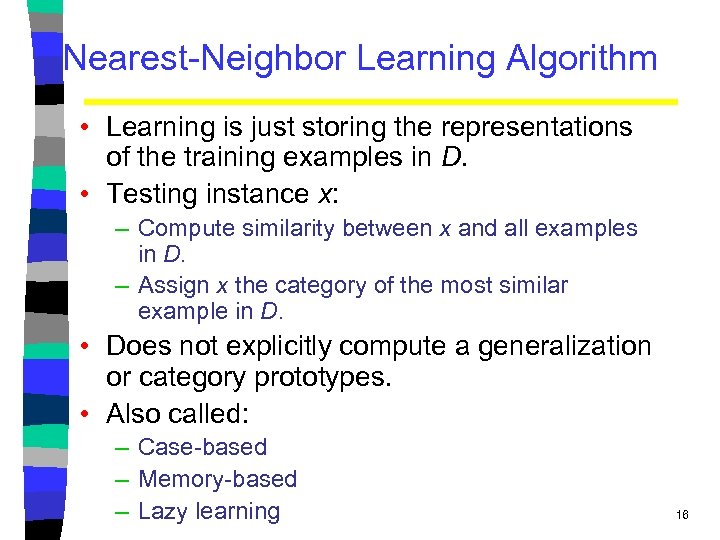Nearest-Neighbor Learning Algorithm • Learning is just storing the representations of the training examples in D. • Testing instance x: – Compute similarity between x and all examples in D. – Assign x the category of the most similar example in D. • Does not explicitly compute a generalization or category prototypes. • Also called: – Case-based – Memory-based – Lazy learning 16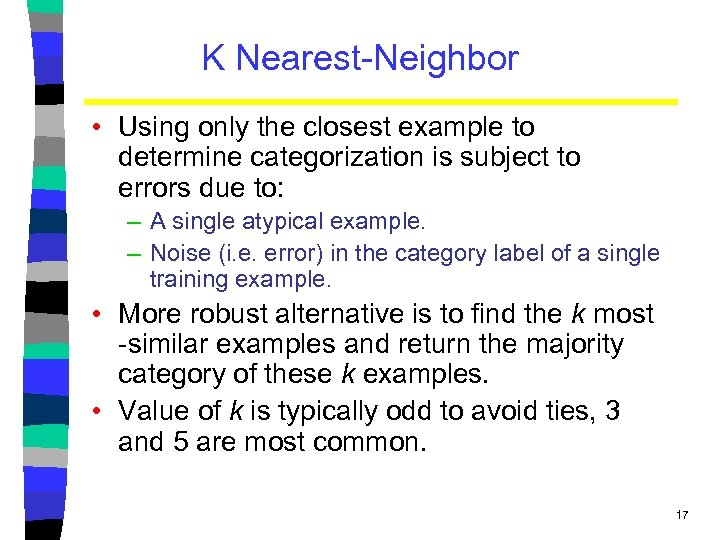K Nearest-Neighbor • Using only the closest example to determine categorization is subject to errors due to: – A single atypical example. – Noise (i. e. error) in the category label of a single training example. • More robust alternative is to find the k most -similar examples and return the majority category of these k examples. • Value of k is typically odd to avoid ties, 3 and 5 are most common. 17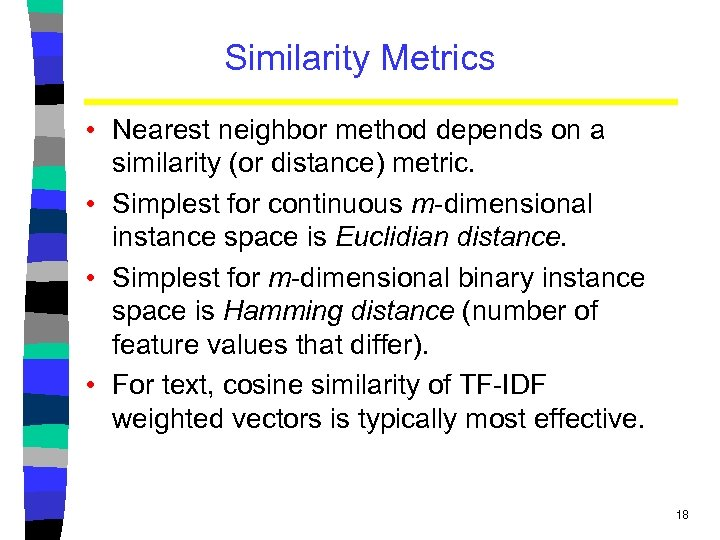Similarity Metrics • Nearest neighbor method depends on a similarity (or distance) metric. • Simplest for continuous m-dimensional instance space is Euclidian distance. • Simplest for m-dimensional binary instance space is Hamming distance (number of feature values that differ). • For text, cosine similarity of TF-IDF weighted vectors is typically most effective. 18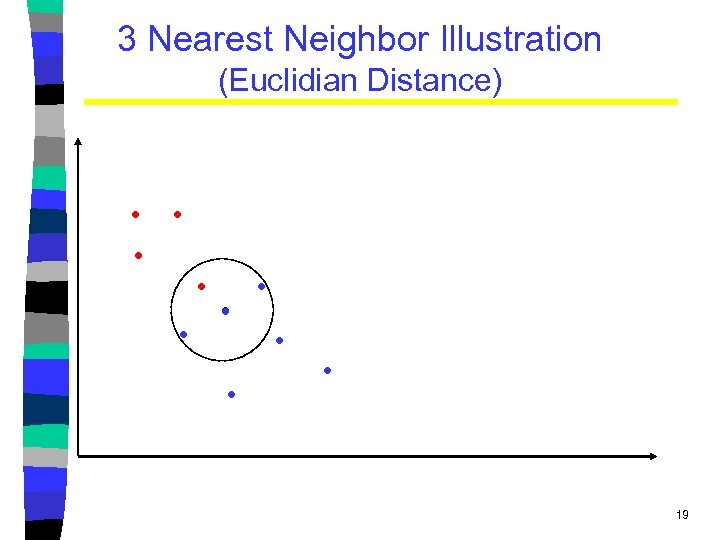3 Nearest Neighbor Illustration (Euclidian Distance) . . 19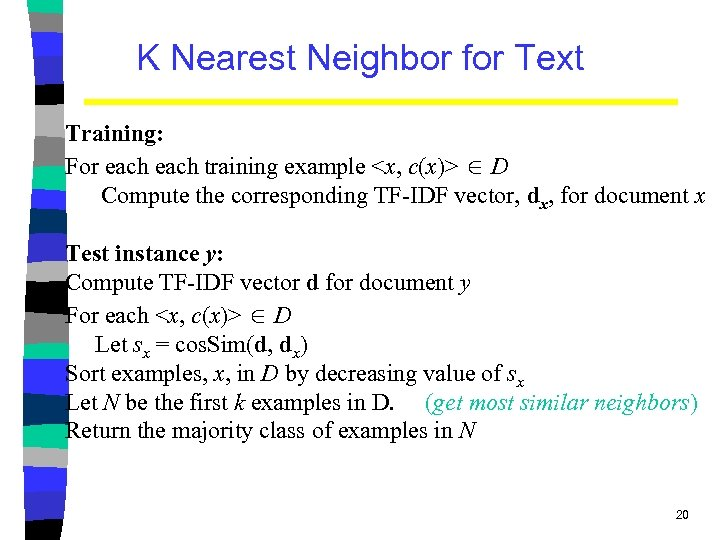K Nearest Neighbor for Text Training: For each training example D Compute the corresponding TF-IDF vector, dx, for document x Test instance y: Compute TF-IDF vector d for document y For each D Let sx = cos. Sim(d, dx) Sort examples, x, in D by decreasing value of sx Let N be the first k examples in D. (get most similar neighbors) Return the majority class of examples in N 20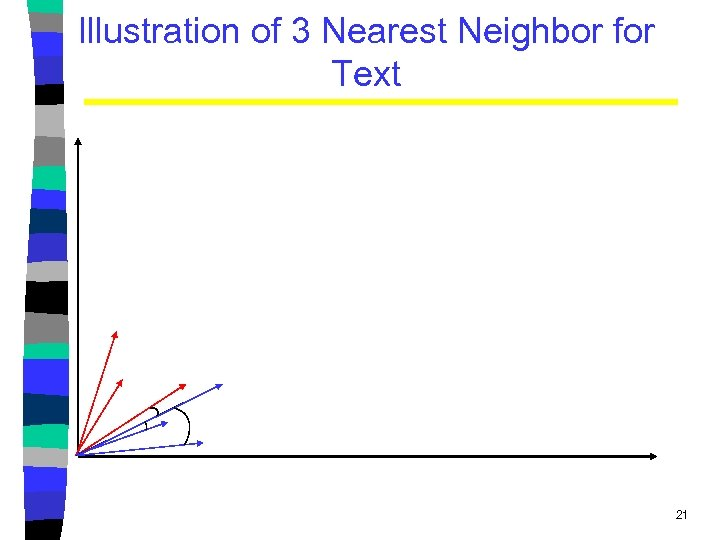Illustration of 3 Nearest Neighbor for Text 21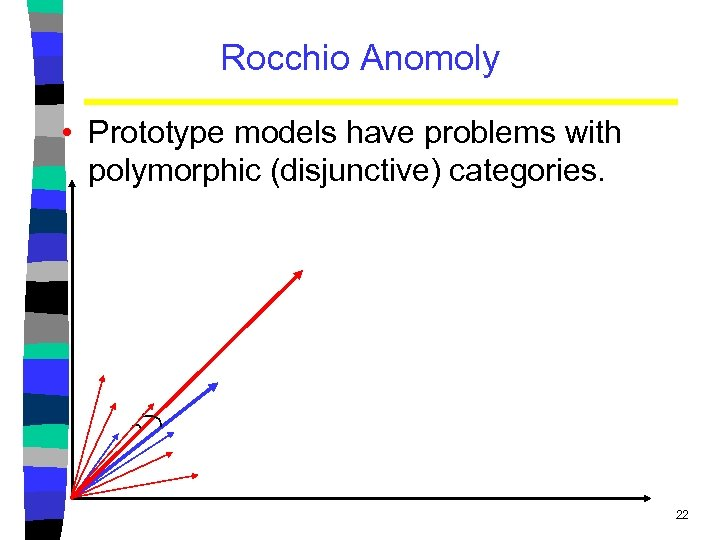Rocchio Anomoly • Prototype models have problems with polymorphic (disjunctive) categories. 22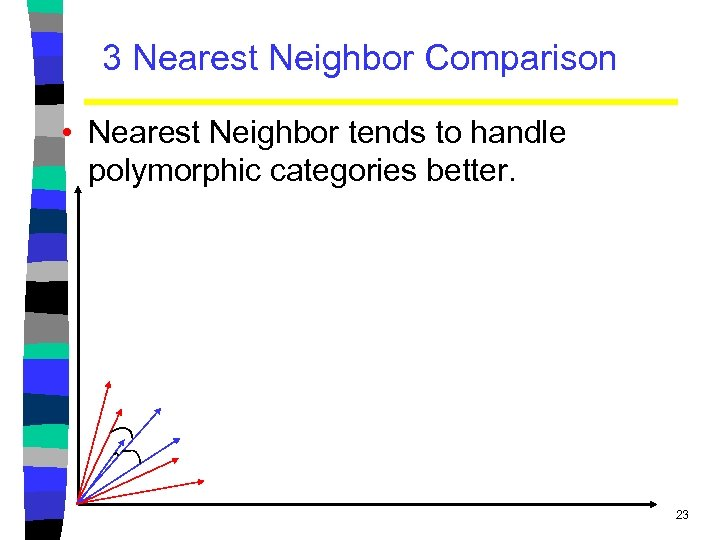3 Nearest Neighbor Comparison • Nearest Neighbor tends to handle polymorphic categories better. 23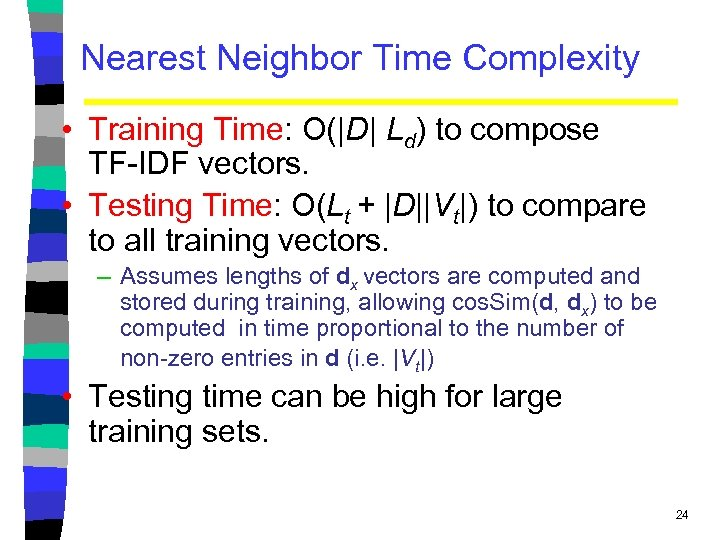Nearest Neighbor Time Complexity • Training Time: O(|D| Ld) to compose TF-IDF vectors. • Testing Time: O(Lt + |D||Vt|) to compare to all training vectors. – Assumes lengths of dx vectors are computed and stored during training, allowing cos. Sim(d, dx) to be computed in time proportional to the number of non-zero entries in d (i. e. |Vt|) • Testing time can be high for large training sets. 24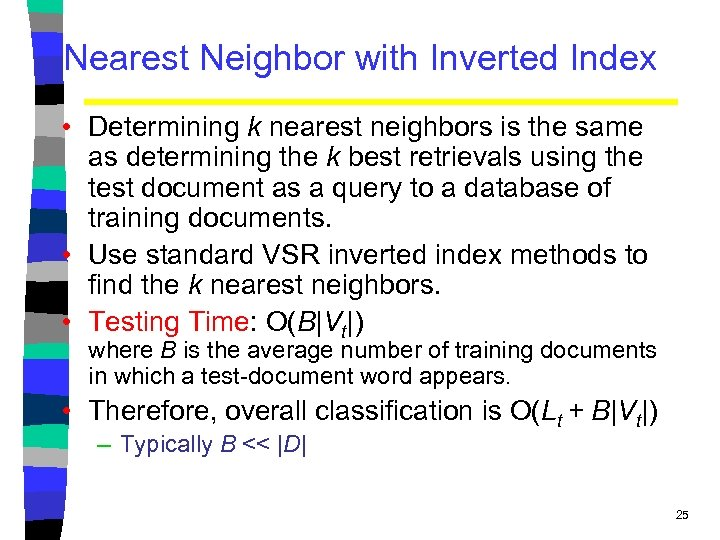Nearest Neighbor with Inverted Index • Determining k nearest neighbors is the same as determining the k best retrievals using the test document as a query to a database of training documents. • Use standard VSR inverted index methods to find the k nearest neighbors. • Testing Time: O(B|Vt|) where B is the average number of training documents in which a test-document word appears. • Therefore, overall classification is O(Lt + B|Vt|) – Typically B << |D| 25Bayesian Methods • Learning and classification methods based on probability theory. • Bayes theorem plays a critical role in probabilistic learning and classification. • Uses prior probability of each category given no information about an item. • Categorization produces a posterior probability distribution over the possible categories given a description of an item. 26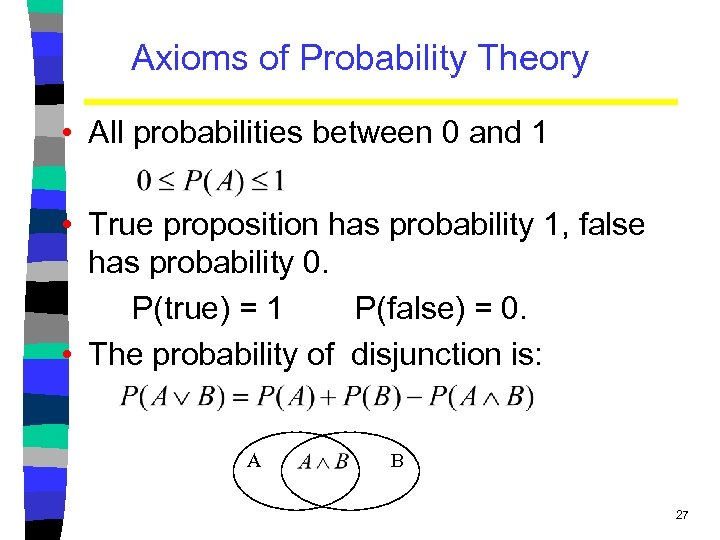Axioms of Probability Theory • All probabilities between 0 and 1 • True proposition has probability 1, false has probability 0. P(true) = 1 P(false) = 0. • The probability of disjunction is: A B 27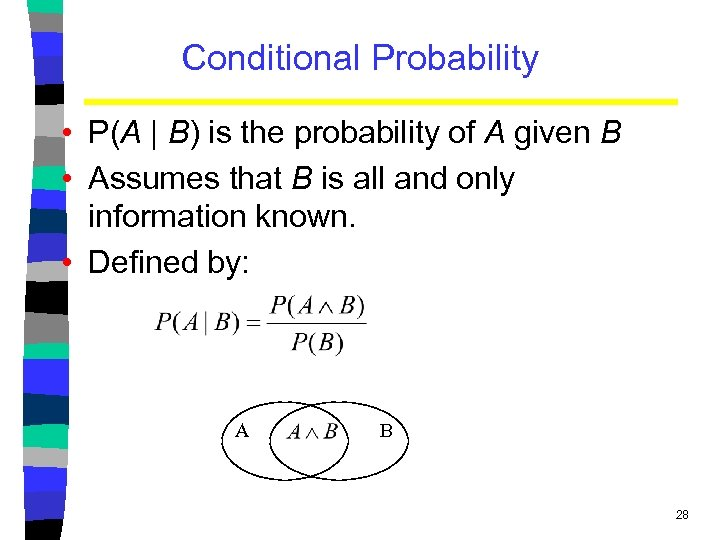Conditional Probability • P(A | B) is the probability of A given B • Assumes that B is all and only information known. • Defined by: A B 28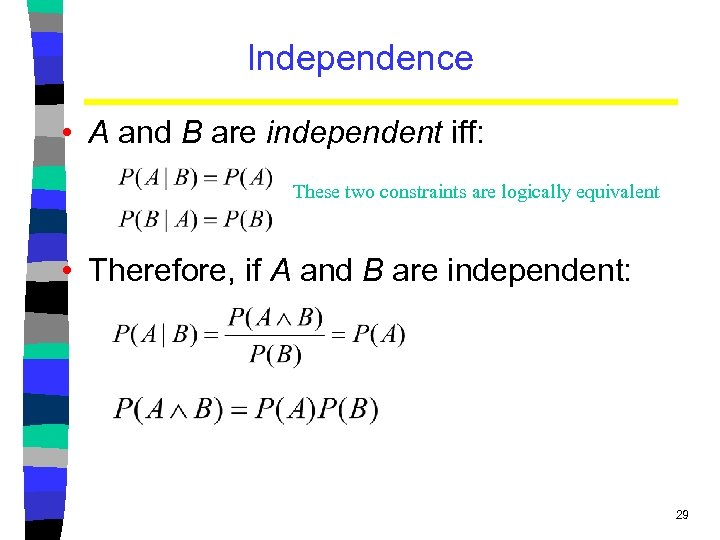Independence • A and B are independent iff: These two constraints are logically equivalent • Therefore, if A and B are independent: 29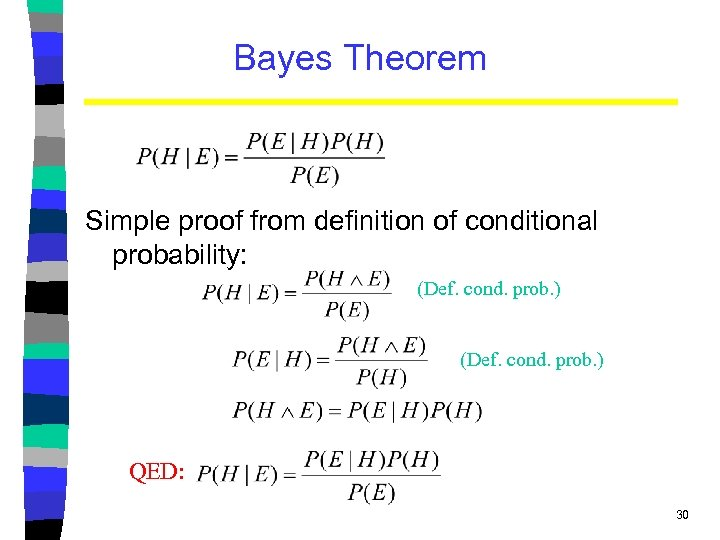Bayes Theorem Simple proof from definition of conditional probability: (Def. cond. prob. ) QED: 30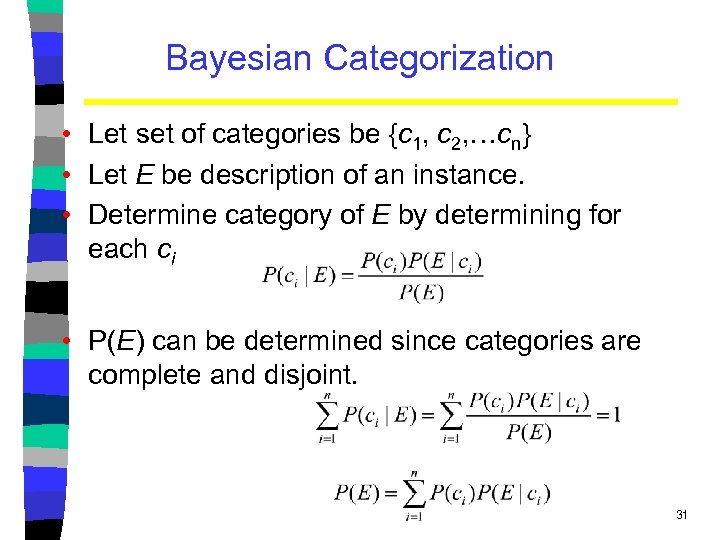Bayesian Categorization • Let set of categories be {c 1, c 2, …cn} • Let E be description of an instance. • Determine category of E by determining for each ci • P(E) can be determined since categories are complete and disjoint. 31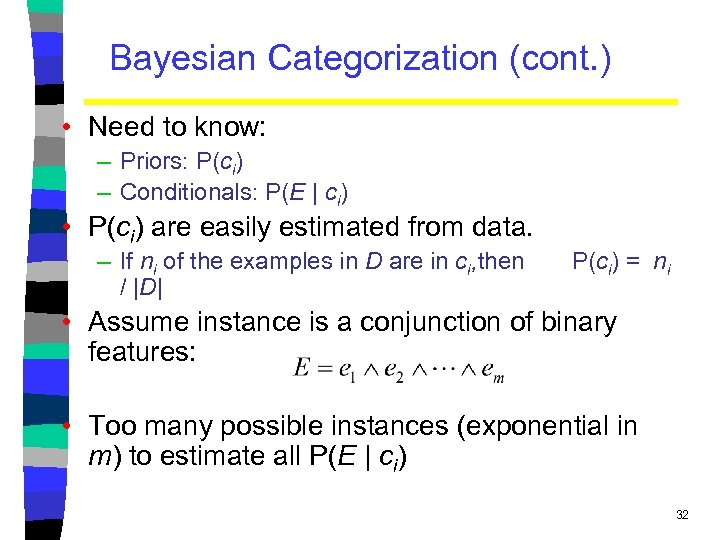Bayesian Categorization (cont. ) • Need to know: – Priors: P(ci) – Conditionals: P(E | ci) • P(ci) are easily estimated from data. – If ni of the examples in D are in ci, then / |D| P(ci) = ni • Assume instance is a conjunction of binary features: • Too many possible instances (exponential in m) to estimate all P(E | ci) 32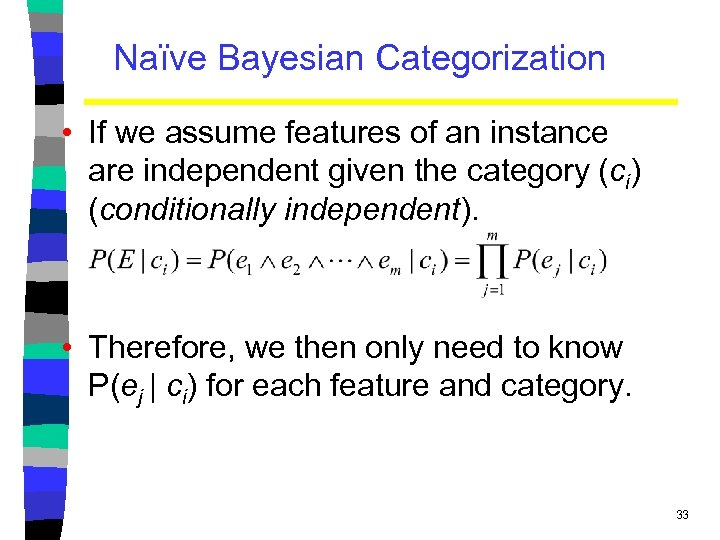Naïve Bayesian Categorization • If we assume features of an instance are independent given the category (ci) (conditionally independent). • Therefore, we then only need to know P(ej | ci) for each feature and category. 33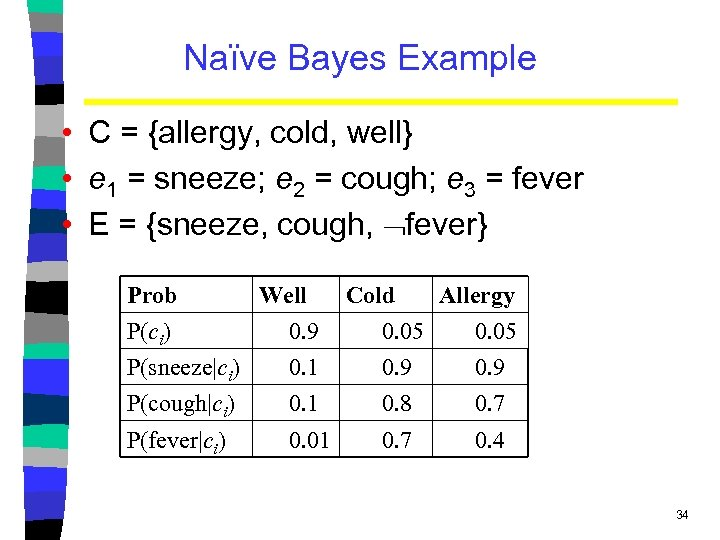Naïve Bayes Example • C = {allergy, cold, well} • e 1 = sneeze; e 2 = cough; e 3 = fever • E = {sneeze, cough, fever} Prob Well Cold Allergy P(ci) 0. 9 0. 05 P(sneeze|ci) 0. 1 0. 9 P(cough|ci) 0. 1 0. 8 0. 7 P(fever|ci) 0. 01 0. 7 0. 4 34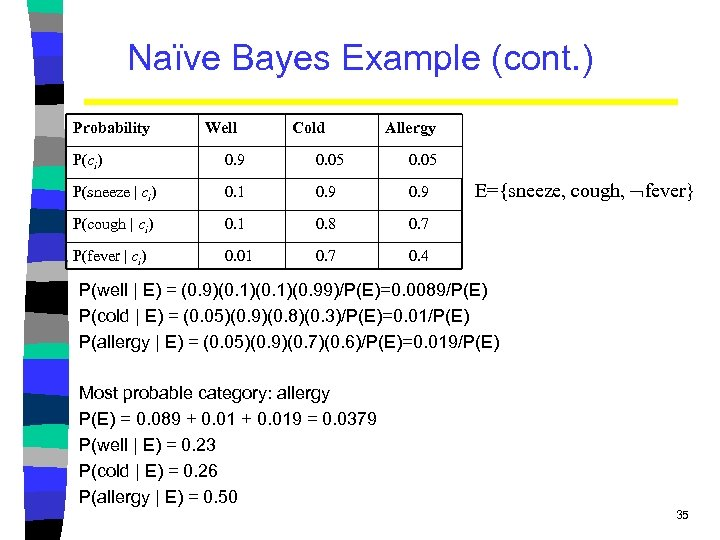Naïve Bayes Example (cont. ) Probability Well Cold Allergy P(ci) 0. 9 0. 05 P(sneeze | ci) 0. 1 0. 9 P(cough | ci) 0. 1 0. 8 0. 7 P(fever | ci) 0. 01 0. 7 0. 4 E={sneeze, cough, fever} P(well | E) = (0. 9)(0. 1)(0. 99)/P(E)=0. 0089/P(E) P(cold | E) = (0. 05)(0. 9)(0. 8)(0. 3)/P(E)=0. 01/P(E) P(allergy | E) = (0. 05)(0. 9)(0. 7)(0. 6)/P(E)=0. 019/P(E) Most probable category: allergy P(E) = 0. 089 + 0. 019 = 0. 0379 P(well | E) = 0. 23 P(cold | E) = 0. 26 P(allergy | E) = 0. 50 35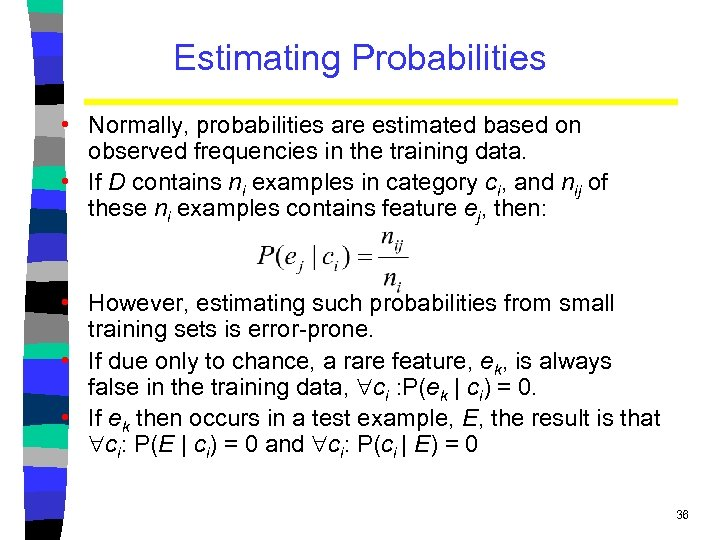Estimating Probabilities • Normally, probabilities are estimated based on observed frequencies in the training data. • If D contains ni examples in category ci, and nij of these ni examples contains feature ej, then: • However, estimating such probabilities from small training sets is error-prone. • If due only to chance, a rare feature, ek, is always false in the training data, ci : P(ek | ci) = 0. • If ek then occurs in a test example, E, the result is that ci: P(E | ci) = 0 and ci: P(ci | E) = 0 36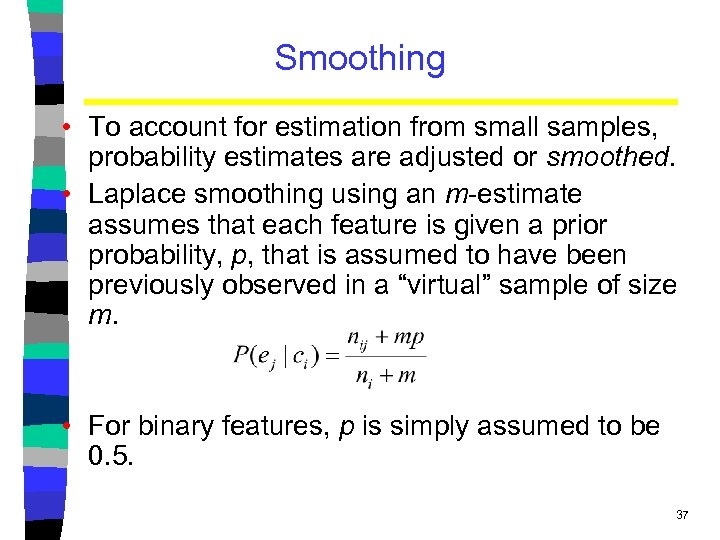Smoothing • To account for estimation from small samples, probability estimates are adjusted or smoothed. • Laplace smoothing using an m-estimate assumes that each feature is given a prior probability, p, that is assumed to have been previously observed in a “virtual” sample of size m. • For binary features, p is simply assumed to be 0. 5. 37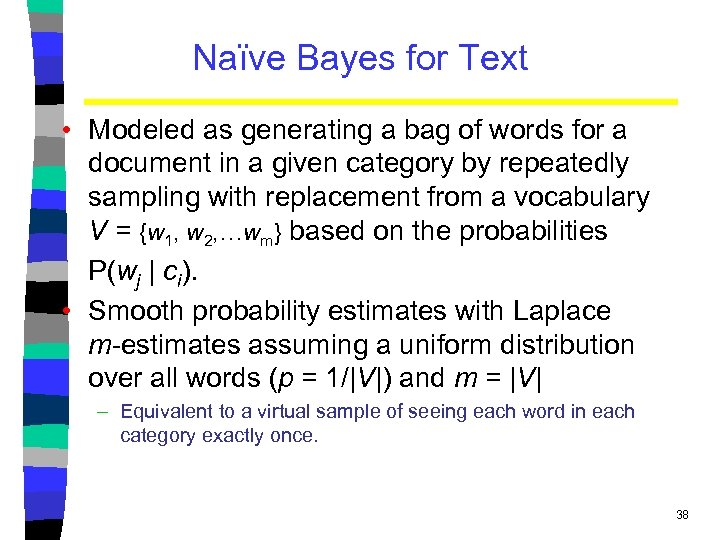Naïve Bayes for Text • Modeled as generating a bag of words for a document in a given category by repeatedly sampling with replacement from a vocabulary V = {w 1, w 2, …wm} based on the probabilities P(wj | ci). • Smooth probability estimates with Laplace m-estimates assuming a uniform distribution over all words (p = 1/|V|) and m = |V| – Equivalent to a virtual sample of seeing each word in each category exactly once. 38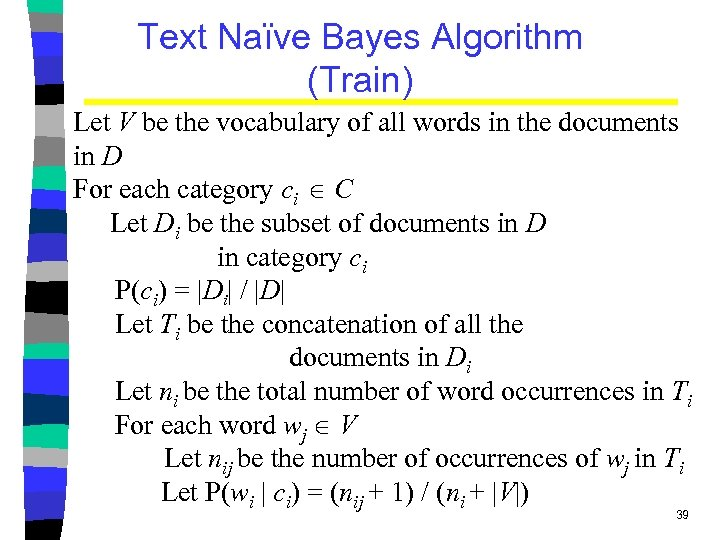Text Naïve Bayes Algorithm (Train) Let V be the vocabulary of all words in the documents in D For each category ci C Let Di be the subset of documents in D in category ci P(ci) = |Di| / |D| Let Ti be the concatenation of all the documents in Di Let ni be the total number of word occurrences in Ti For each word wj V Let nij be the number of occurrences of wj in Ti Let P(wi | ci) = (nij + 1) / (ni + |V|) 39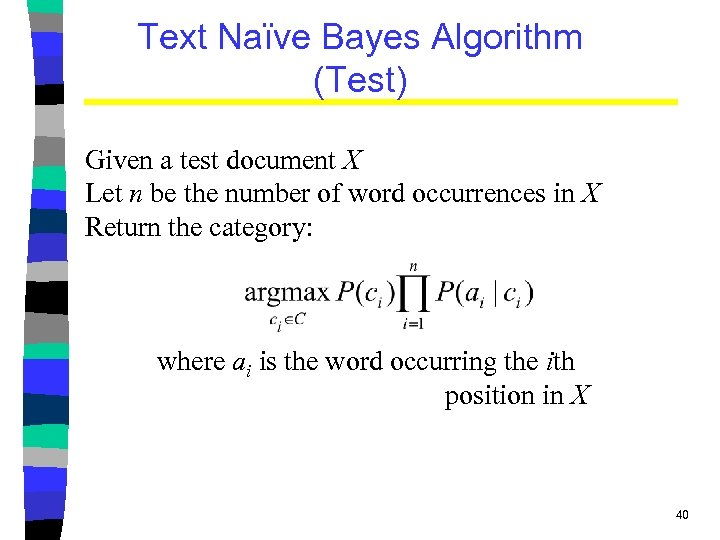Text Naïve Bayes Algorithm (Test) Given a test document X Let n be the number of word occurrences in X Return the category: where ai is the word occurring the ith position in X 40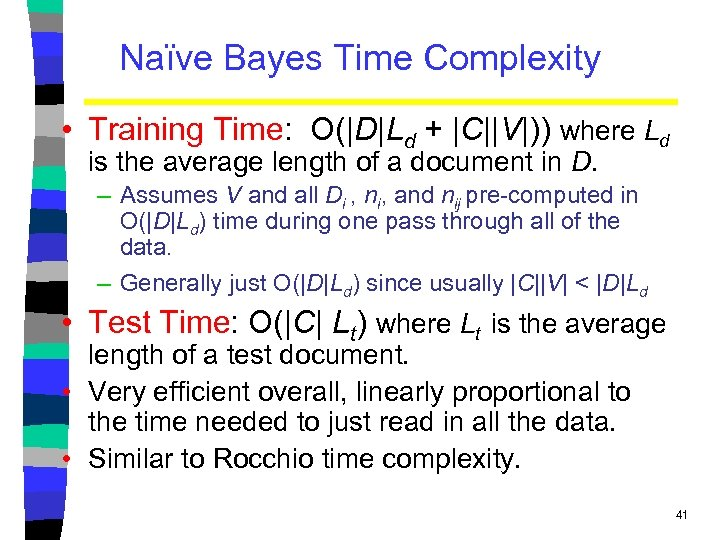Naïve Bayes Time Complexity • Training Time: O(|D|Ld + |C||V|)) where Ld is the average length of a document in D. – Assumes V and all Di , ni, and nij pre-computed in O(|D|Ld) time during one pass through all of the data. – Generally just O(|D|Ld) since usually |C||V| < |D|Ld • Test Time: O(|C| Lt) where Lt is the average length of a test document. • Very efficient overall, linearly proportional to the time needed to just read in all the data. • Similar to Rocchio time complexity. 41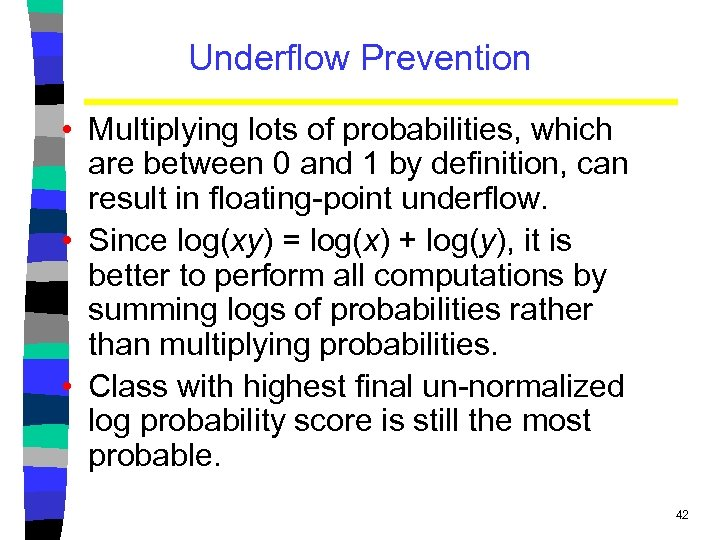Underflow Prevention • Multiplying lots of probabilities, which are between 0 and 1 by definition, can result in floating-point underflow. • Since log(xy) = log(x) + log(y), it is better to perform all computations by summing logs of probabilities rather than multiplying probabilities. • Class with highest final un-normalized log probability score is still the most probable. 42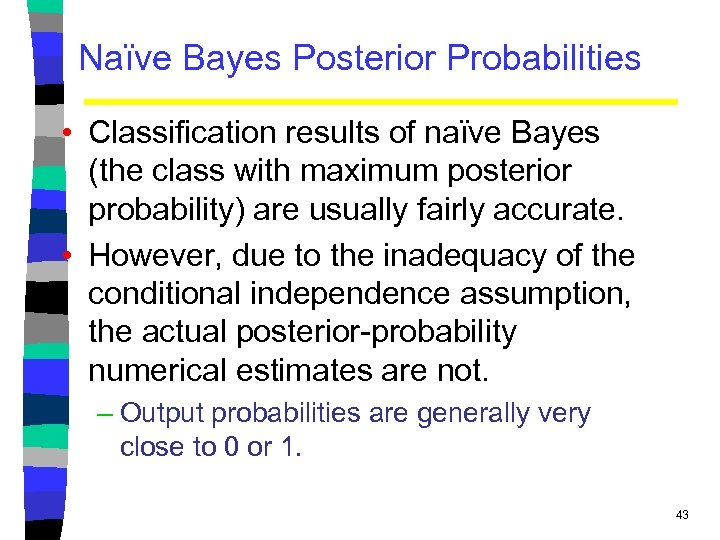Naïve Bayes Posterior Probabilities • Classification results of naïve Bayes (the class with maximum posterior probability) are usually fairly accurate. • However, due to the inadequacy of the conditional independence assumption, the actual posterior-probability numerical estimates are not. – Output probabilities are generally very close to 0 or 1. 43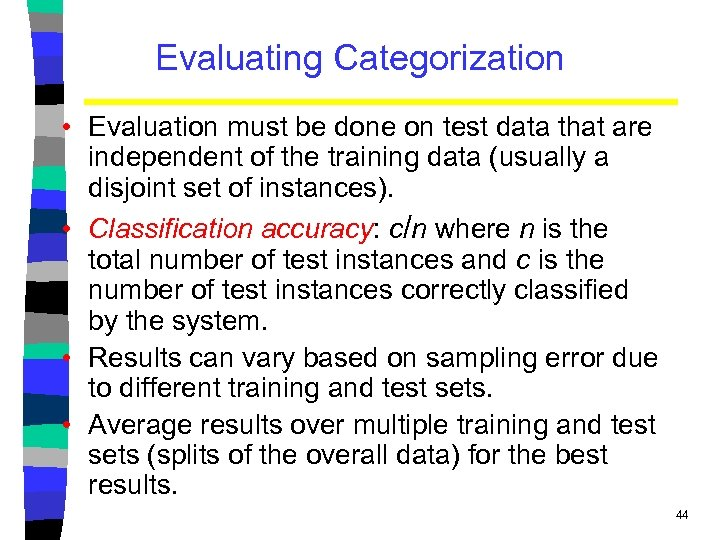Evaluating Categorization • Evaluation must be done on test data that are independent of the training data (usually a disjoint set of instances). • Classification accuracy: c/n where n is the total number of test instances and c is the number of test instances correctly classified by the system. • Results can vary based on sampling error due to different training and test sets. • Average results over multiple training and test sets (splits of the overall data) for the best results. 44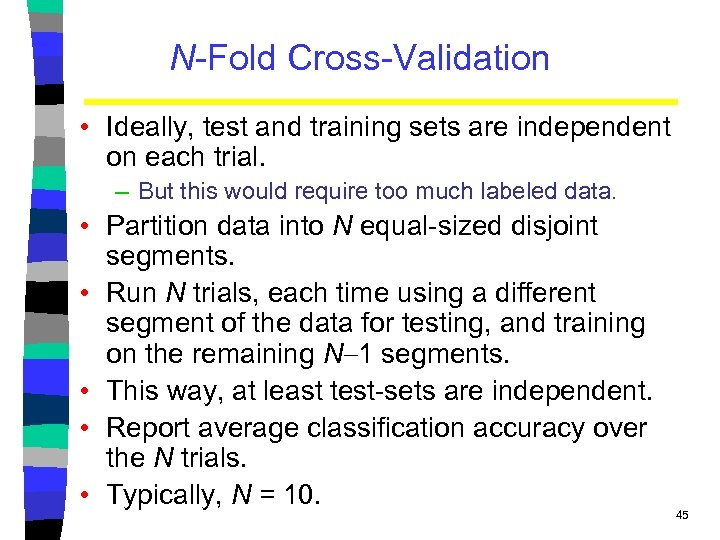N-Fold Cross-Validation • Ideally, test and training sets are independent on each trial. – But this would require too much labeled data. • Partition data into N equal-sized disjoint segments. • Run N trials, each time using a different segment of the data for testing, and training on the remaining N 1 segments. • This way, at least test-sets are independent. • Report average classification accuracy over the N trials. • Typically, N = 10. 45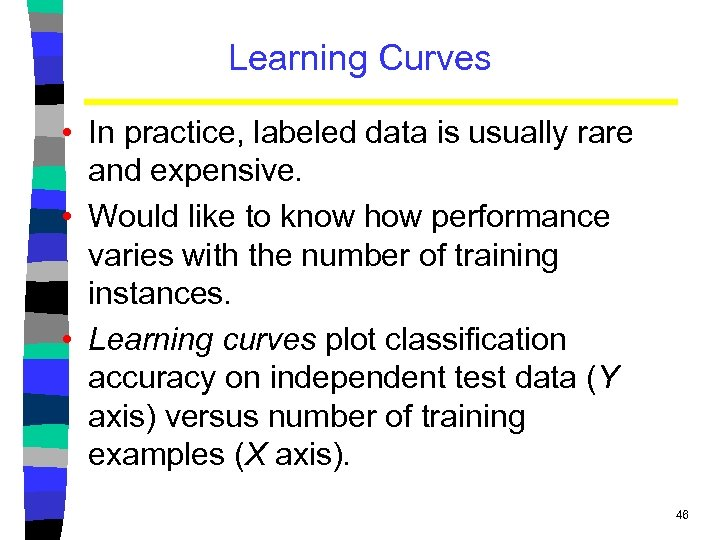Learning Curves • In practice, labeled data is usually rare and expensive. • Would like to know how performance varies with the number of training instances. • Learning curves plot classification accuracy on independent test data (Y axis) versus number of training examples (X axis). 46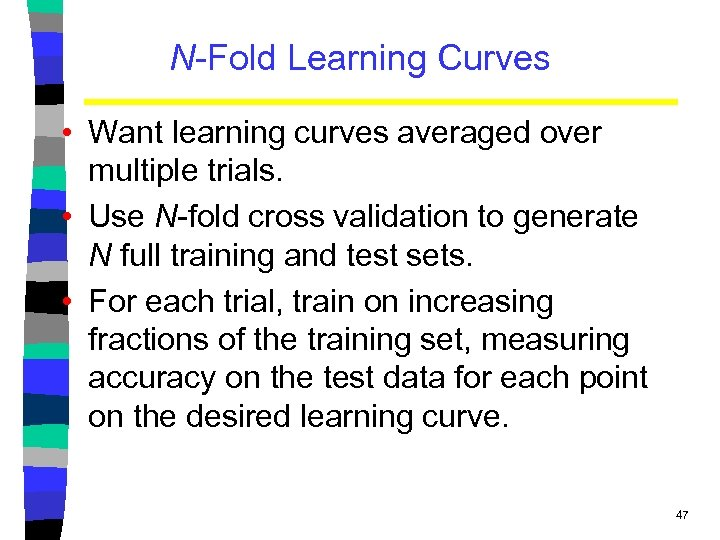N-Fold Learning Curves • Want learning curves averaged over multiple trials. • Use N-fold cross validation to generate N full training and test sets. • For each trial, train on increasing fractions of the training set, measuring accuracy on the test data for each point on the desired learning curve. 47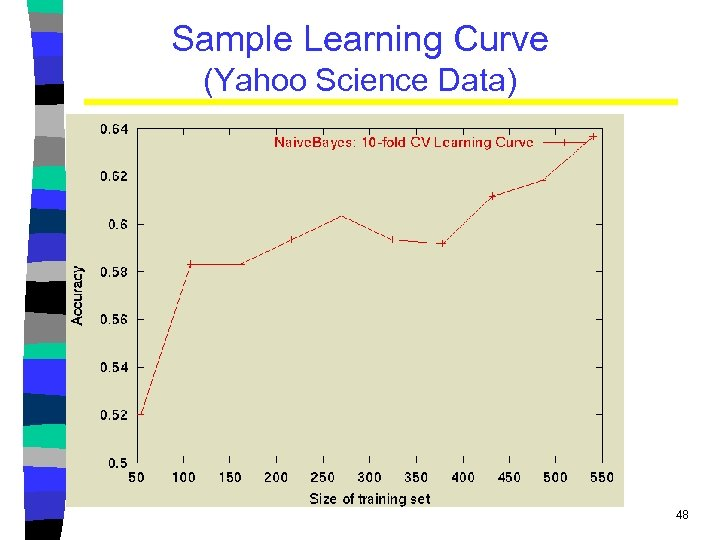Sample Learning Curve (Yahoo Science Data) 48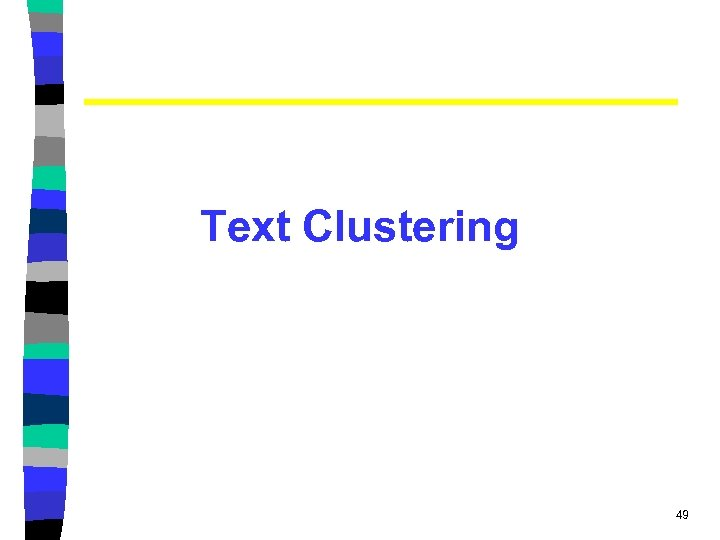Text Clustering 49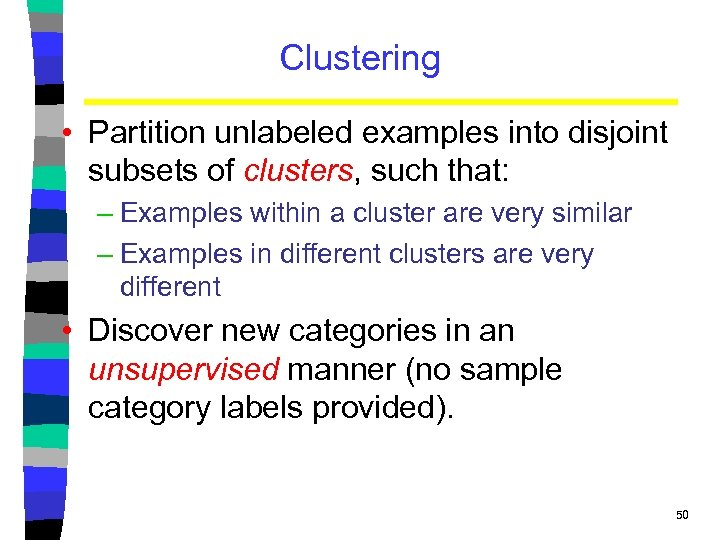Clustering • Partition unlabeled examples into disjoint subsets of clusters, such that: – Examples within a cluster are very similar – Examples in different clusters are very different • Discover new categories in an unsupervised manner (no sample category labels provided). 50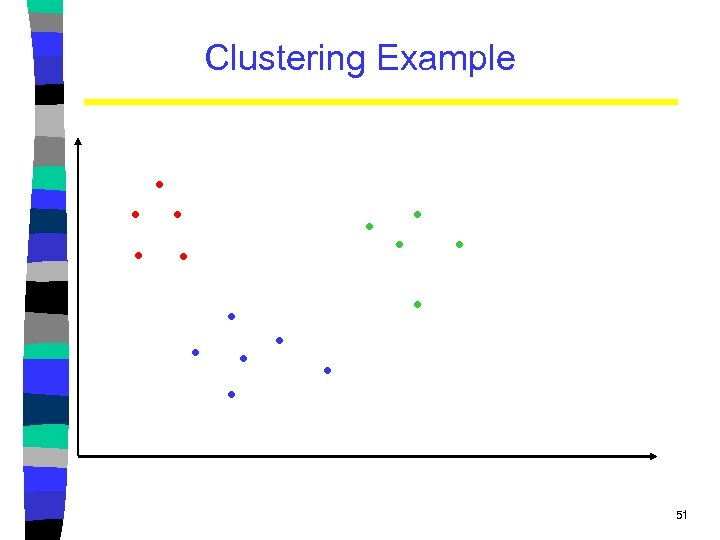Clustering Example . . . . 51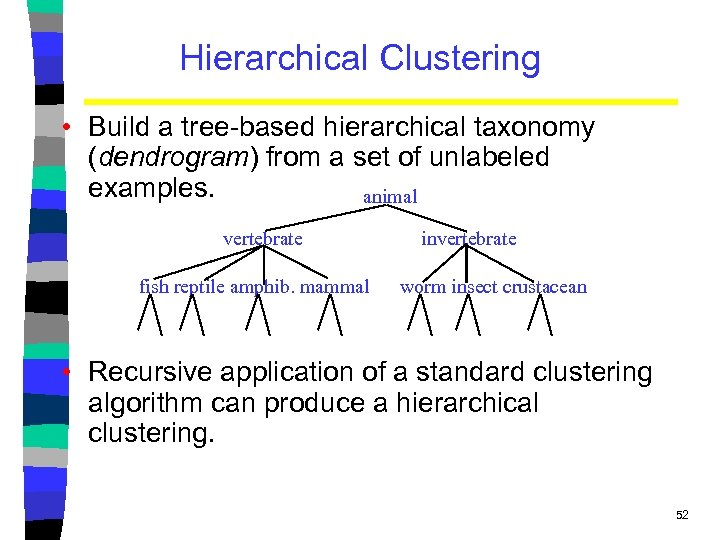Hierarchical Clustering • Build a tree-based hierarchical taxonomy (dendrogram) from a set of unlabeled examples. animal vertebrate fish reptile amphib. mammal invertebrate worm insect crustacean • Recursive application of a standard clustering algorithm can produce a hierarchical clustering. 52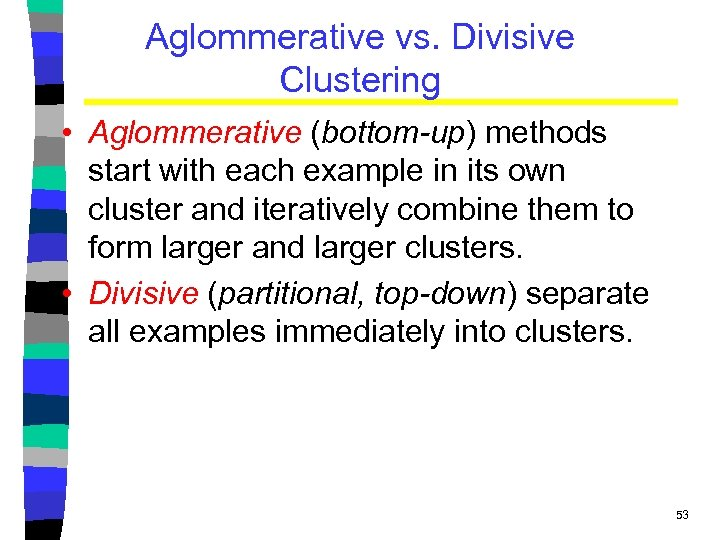Aglommerative vs. Divisive Clustering • Aglommerative (bottom-up) methods start with each example in its own cluster and iteratively combine them to form larger and larger clusters. • Divisive (partitional, top-down) separate all examples immediately into clusters. 53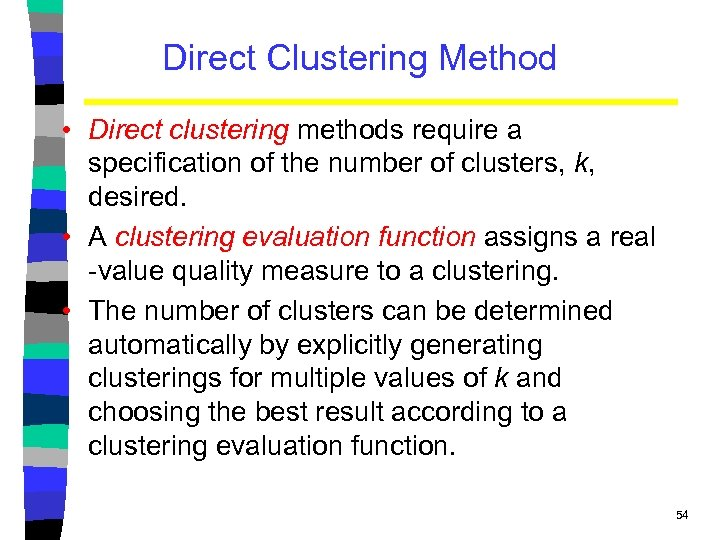Direct Clustering Method • Direct clustering methods require a specification of the number of clusters, k, desired. • A clustering evaluation function assigns a real -value quality measure to a clustering. • The number of clusters can be determined automatically by explicitly generating clusterings for multiple values of k and choosing the best result according to a clustering evaluation function. 54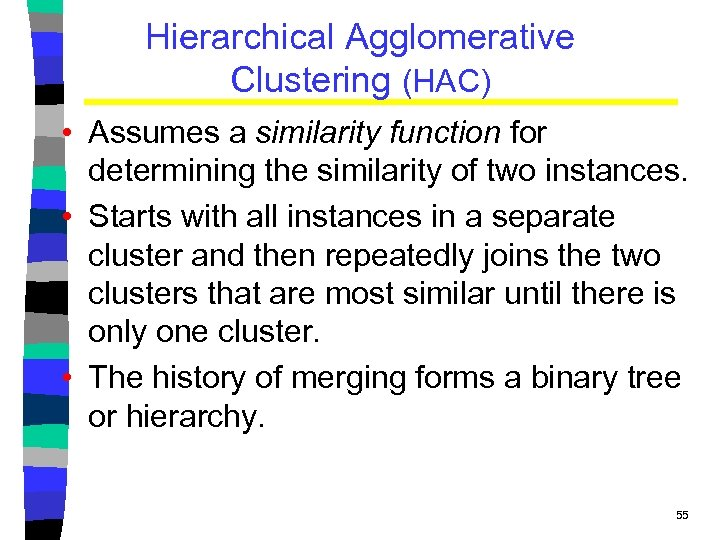Hierarchical Agglomerative Clustering (HAC) • Assumes a similarity function for determining the similarity of two instances. • Starts with all instances in a separate cluster and then repeatedly joins the two clusters that are most similar until there is only one cluster. • The history of merging forms a binary tree or hierarchy. 55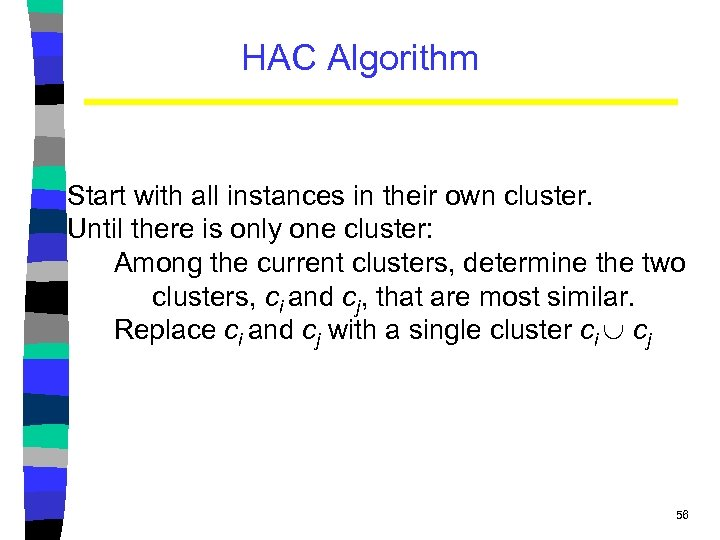HAC Algorithm Start with all instances in their own cluster. Until there is only one cluster: Among the current clusters, determine the two clusters, ci and cj, that are most similar. Replace ci and cj with a single cluster ci cj 56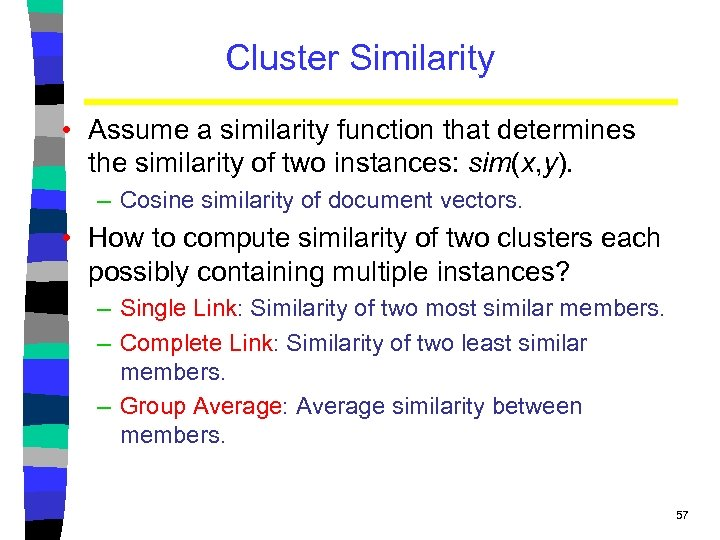Cluster Similarity • Assume a similarity function that determines the similarity of two instances: sim(x, y). – Cosine similarity of document vectors. • How to compute similarity of two clusters each possibly containing multiple instances? – Single Link: Similarity of two most similar members. – Complete Link: Similarity of two least similar members. – Group Average: Average similarity between members. 57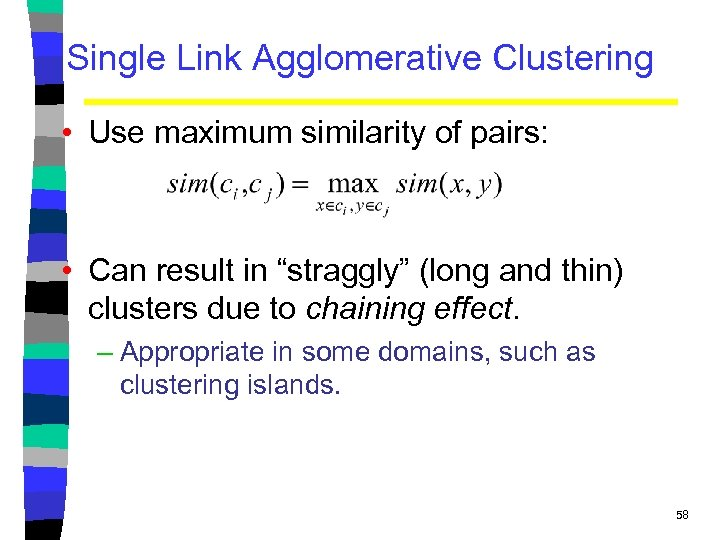Single Link Agglomerative Clustering • Use maximum similarity of pairs: • Can result in “straggly” (long and thin) clusters due to chaining effect. – Appropriate in some domains, such as clustering islands. 58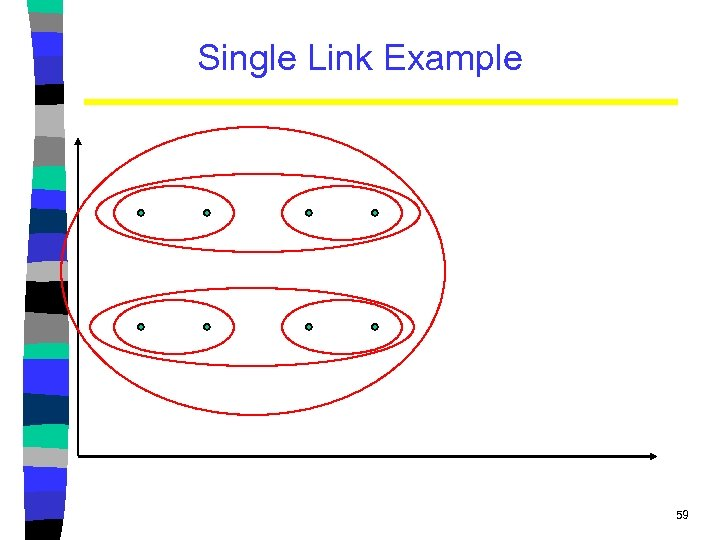Single Link Example 59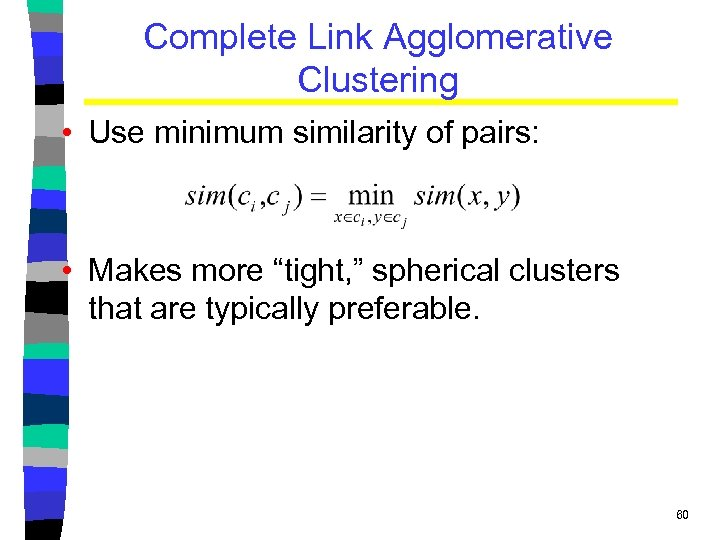Complete Link Agglomerative Clustering • Use minimum similarity of pairs: • Makes more “tight, ” spherical clusters that are typically preferable. 60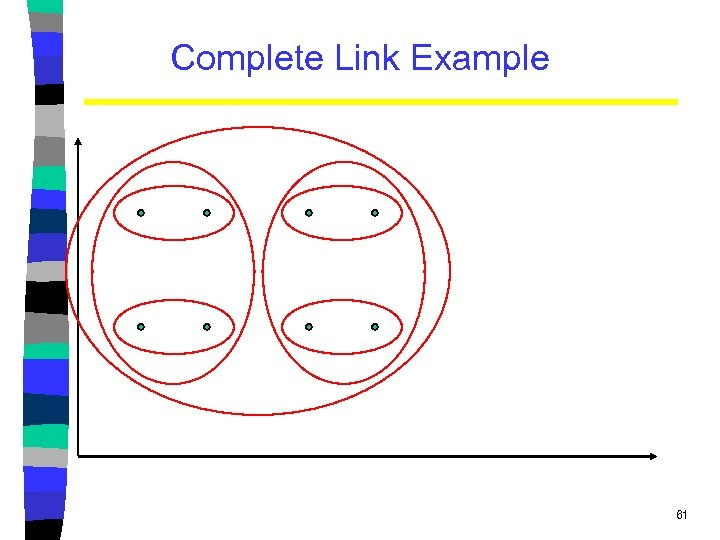Complete Link Example 61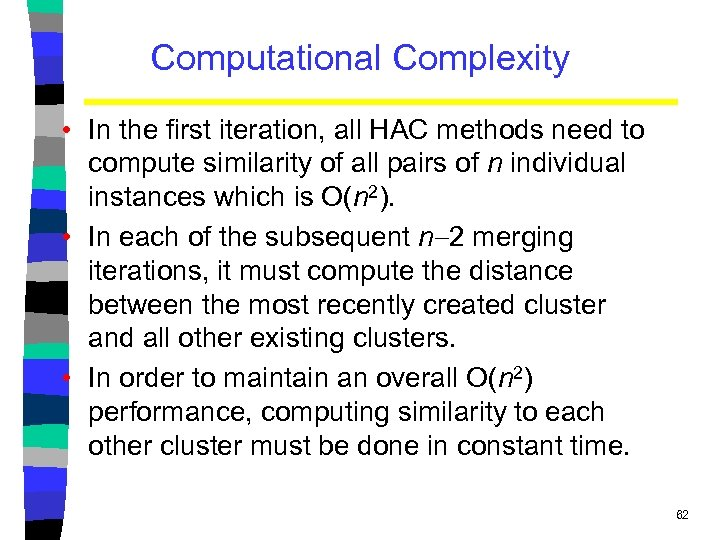Computational Complexity • In the first iteration, all HAC methods need to compute similarity of all pairs of n individual instances which is O(n 2). • In each of the subsequent n 2 merging iterations, it must compute the distance between the most recently created cluster and all other existing clusters. • In order to maintain an overall O(n 2) performance, computing similarity to each other cluster must be done in constant time. 62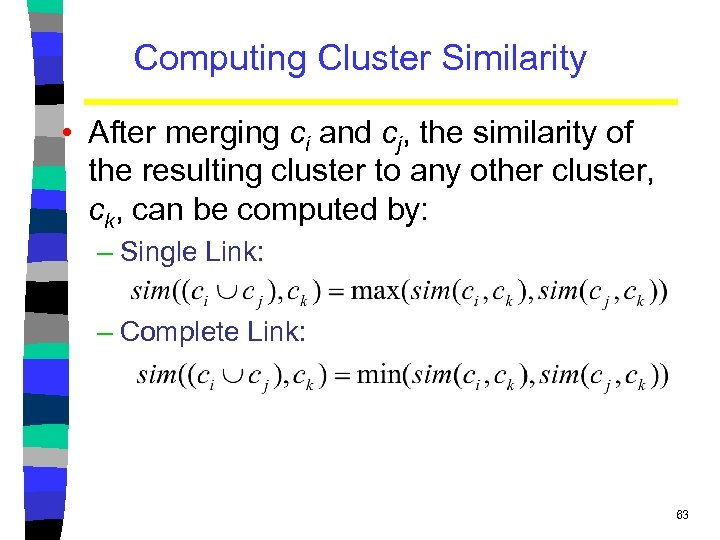Computing Cluster Similarity • After merging ci and cj, the similarity of the resulting cluster to any other cluster, ck, can be computed by: – Single Link: – Complete Link: 63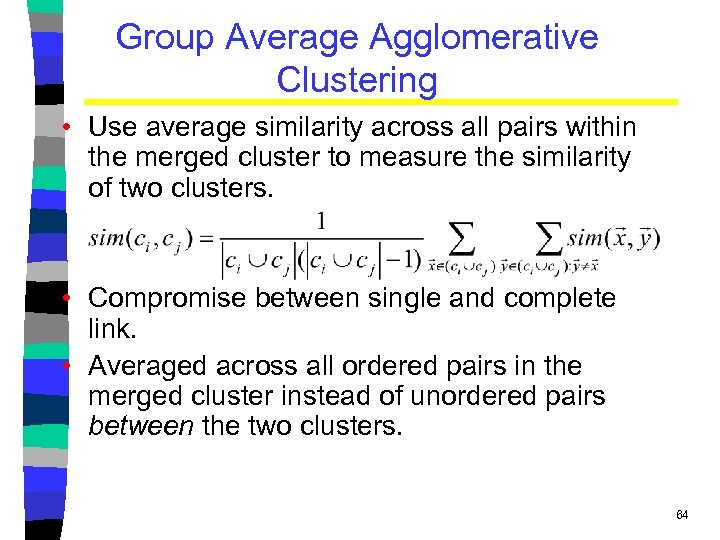Group Average Agglomerative Clustering • Use average similarity across all pairs within the merged cluster to measure the similarity of two clusters. • Compromise between single and complete link. • Averaged across all ordered pairs in the merged cluster instead of unordered pairs between the two clusters. 64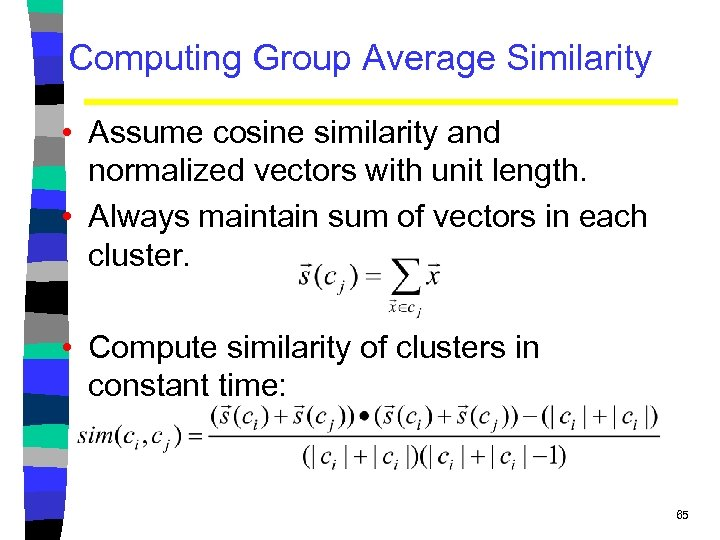Computing Group Average Similarity • Assume cosine similarity and normalized vectors with unit length. • Always maintain sum of vectors in each cluster. • Compute similarity of clusters in constant time: 65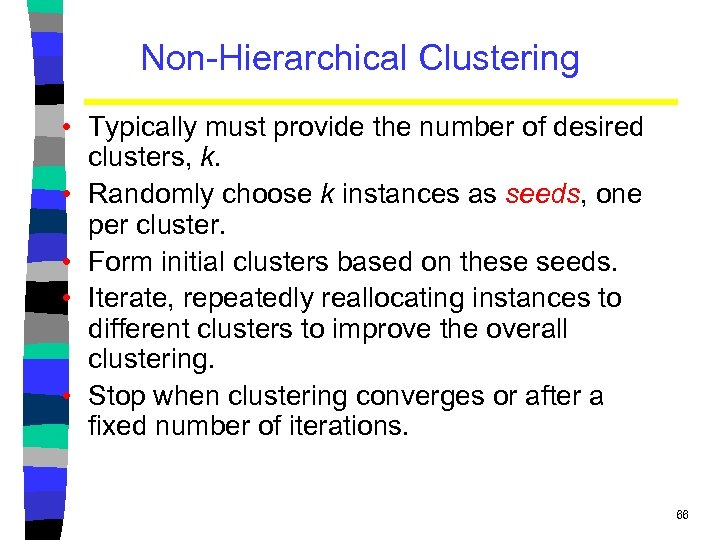Non-Hierarchical Clustering • Typically must provide the number of desired clusters, k. • Randomly choose k instances as seeds, one per cluster. • Form initial clusters based on these seeds. • Iterate, repeatedly reallocating instances to different clusters to improve the overall clustering. • Stop when clustering converges or after a fixed number of iterations. 66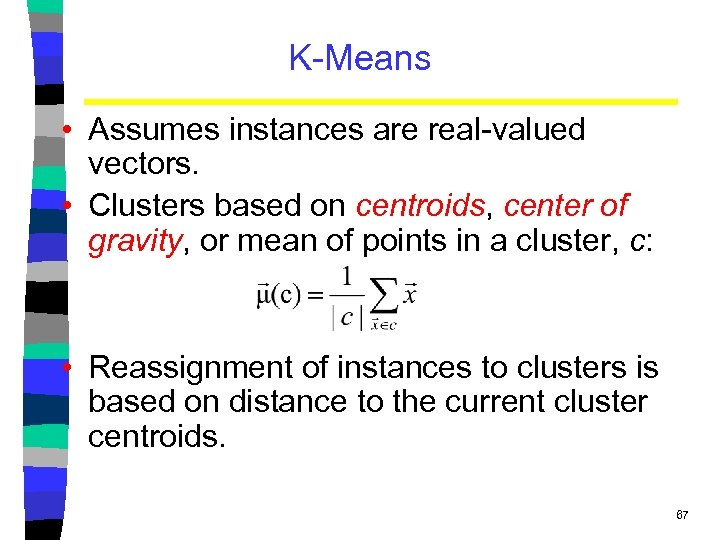K-Means • Assumes instances are real-valued vectors. • Clusters based on centroids, center of gravity, or mean of points in a cluster, c: • Reassignment of instances to clusters is based on distance to the current cluster centroids. 67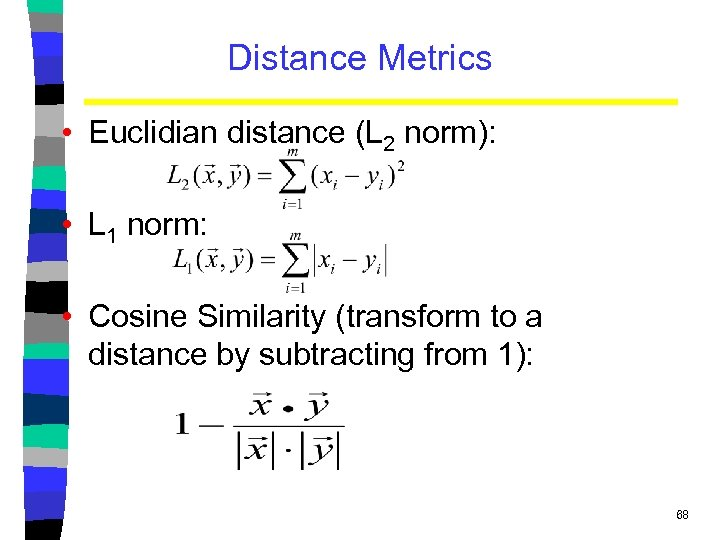Distance Metrics • Euclidian distance (L 2 norm): • L 1 norm: • Cosine Similarity (transform to a distance by subtracting from 1): 68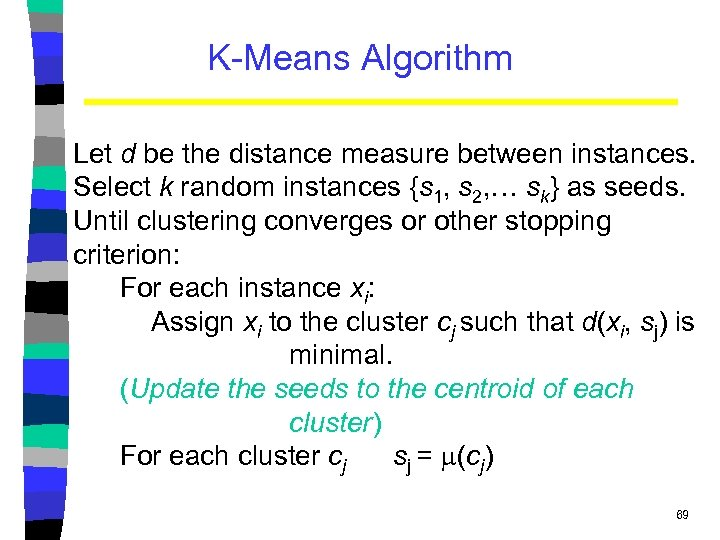K-Means Algorithm Let d be the distance measure between instances. Select k random instances {s 1, s 2, … sk} as seeds. Until clustering converges or other stopping criterion: For each instance xi: Assign xi to the cluster cj such that d(xi, sj) is minimal. (Update the seeds to the centroid of each cluster) For each cluster cj sj = (cj) 69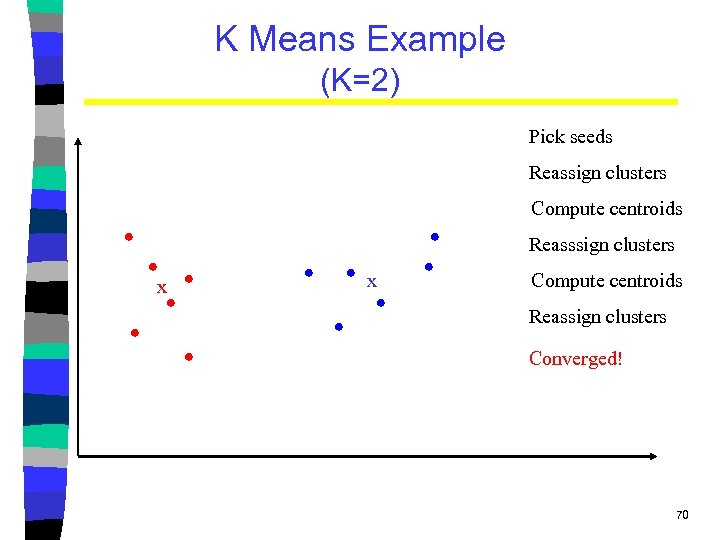K Means Example (K=2) Pick seeds Reassign clusters Compute centroids Reasssign clusters x x Compute centroids Reassign clusters Converged! 70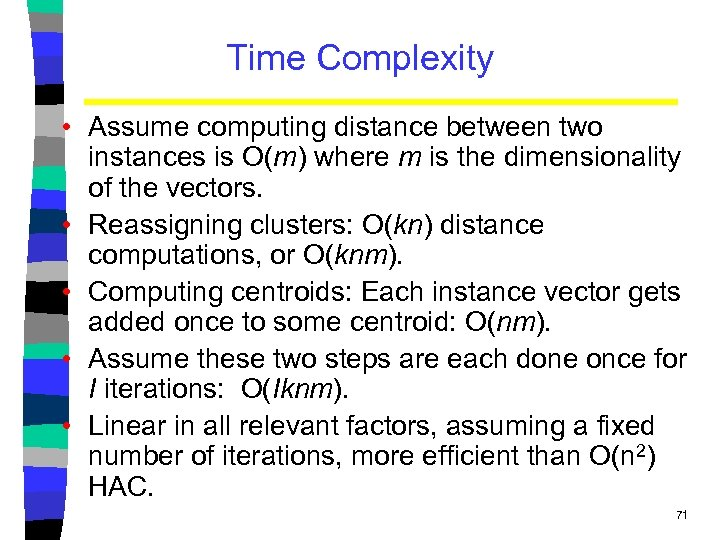Time Complexity • Assume computing distance between two instances is O(m) where m is the dimensionality of the vectors. • Reassigning clusters: O(kn) distance computations, or O(knm). • Computing centroids: Each instance vector gets added once to some centroid: O(nm). • Assume these two steps are each done once for I iterations: O(Iknm). • Linear in all relevant factors, assuming a fixed number of iterations, more efficient than O(n 2) HAC. 71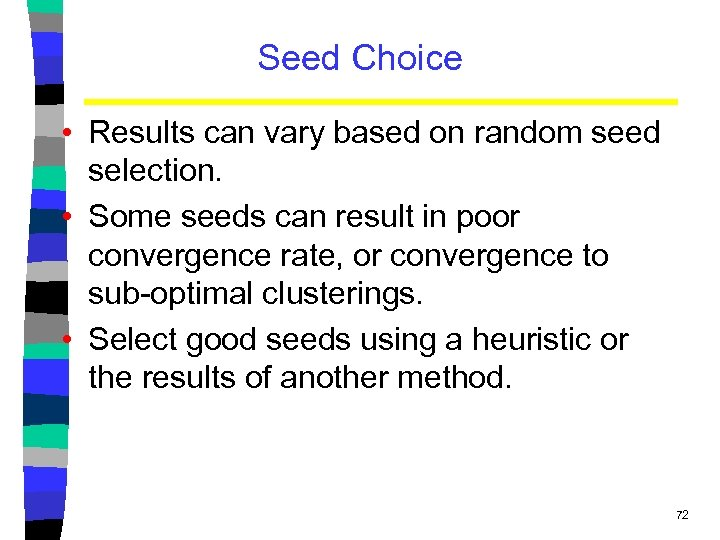Seed Choice • Results can vary based on random seed selection. • Some seeds can result in poor convergence rate, or convergence to sub-optimal clusterings. • Select good seeds using a heuristic or the results of another method. 72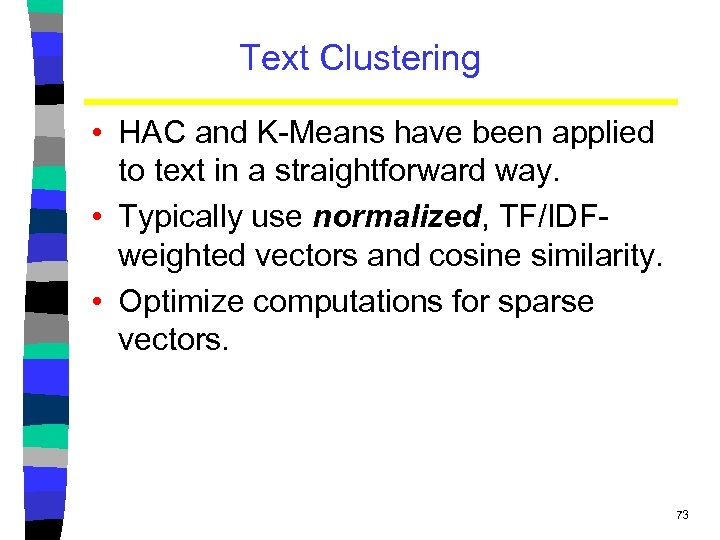Text Clustering • HAC and K-Means have been applied to text in a straightforward way. • Typically use normalized, TF/IDFweighted vectors and cosine similarity. • Optimize computations for sparse vectors. 73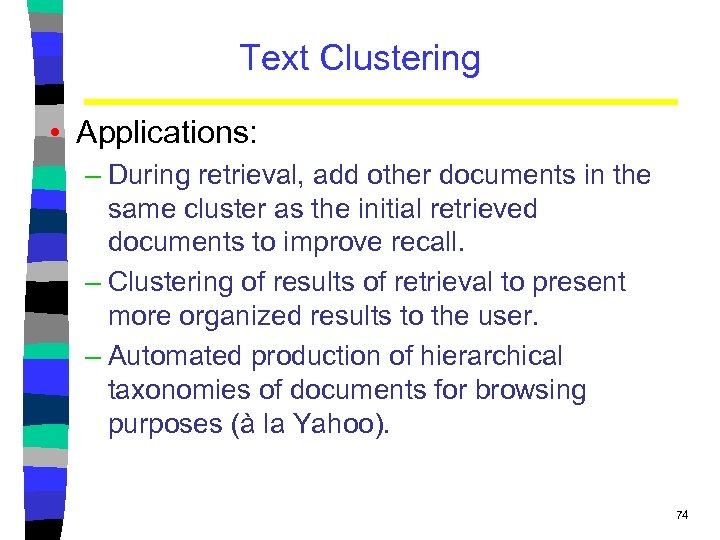Text Clustering • Applications: – During retrieval, add other documents in the same cluster as the initial retrieved documents to improve recall. – Clustering of results of retrieval to present more organized results to the user. – Automated production of hierarchical taxonomies of documents for browsing purposes (à la Yahoo). 74